# Telling the Time Quarter Past Half Past and

• Slides: 10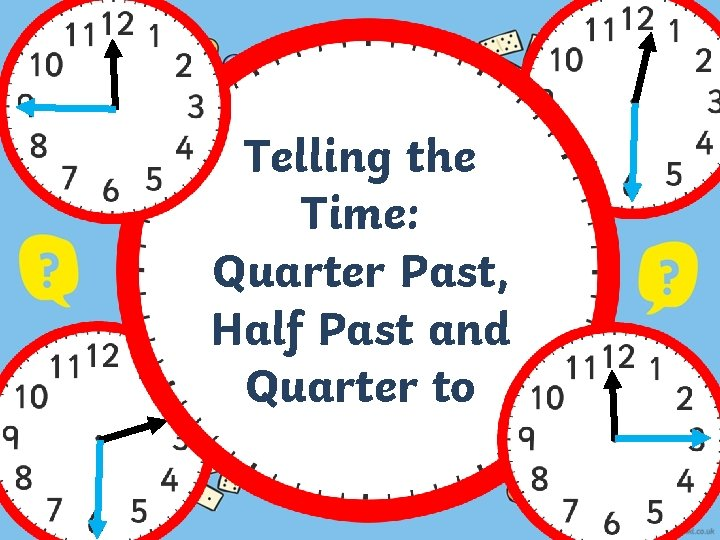Telling the Time: Quarter Past, Half Past and Quarter to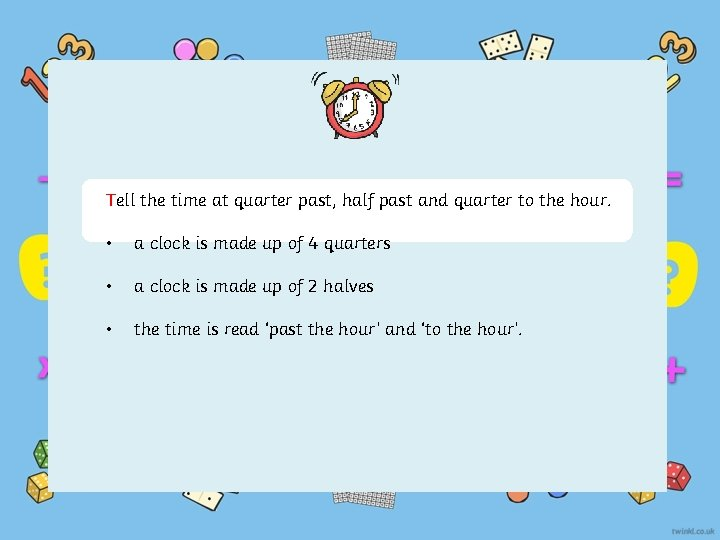Tell the time at quarter past, half past and quarter to the hour. • a clock is made up of 4 quarters • a clock is made up of 2 halves • the time is read ‘past the hour’ and ‘to the hour’.Questions How many seconds are there in a minute? 60 How many minutes are there in an hour? 60 How many minutes are there in quarter of an hour? 15 How many minutes are there in half an hour? 30 How many days are there in a month? 28 -31 How many days are there in a year? 365 How many days are there in a leap year? 366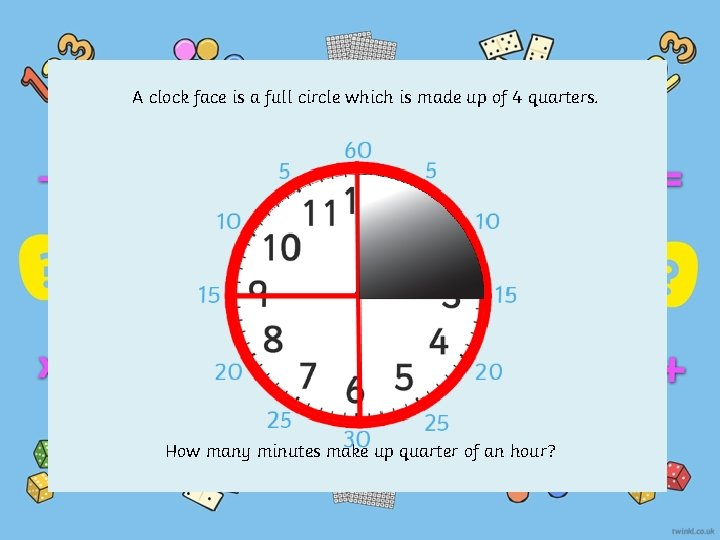A clock face is a full circle which is made up of 4 quarters. How many minutes make up quarter of an hour?There are 15 minutes in quarter of an hour.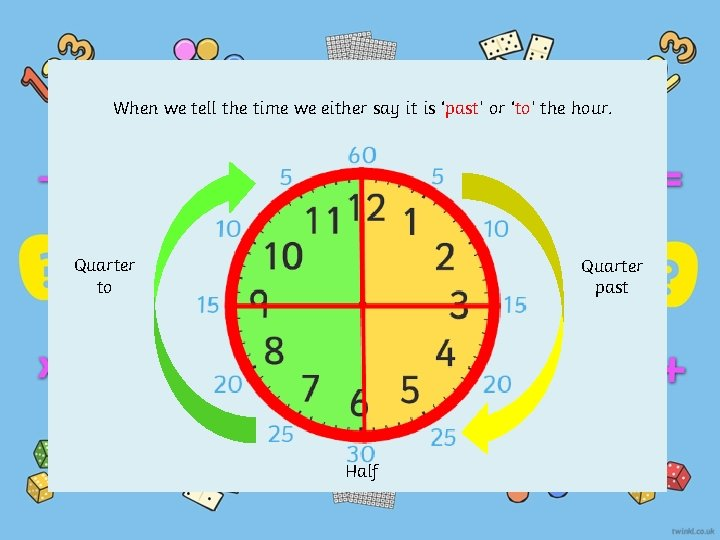When we tell the time we either say it is ‘past’ or ‘to’ the hour. Quarter to Quarter past HalfLook at this time. The big hand is pointing at 3, which is the first quarter. Where is the small hand pointing? Is it on the number? It’s actually a quarter of the way between 1 and 2. Quarter Half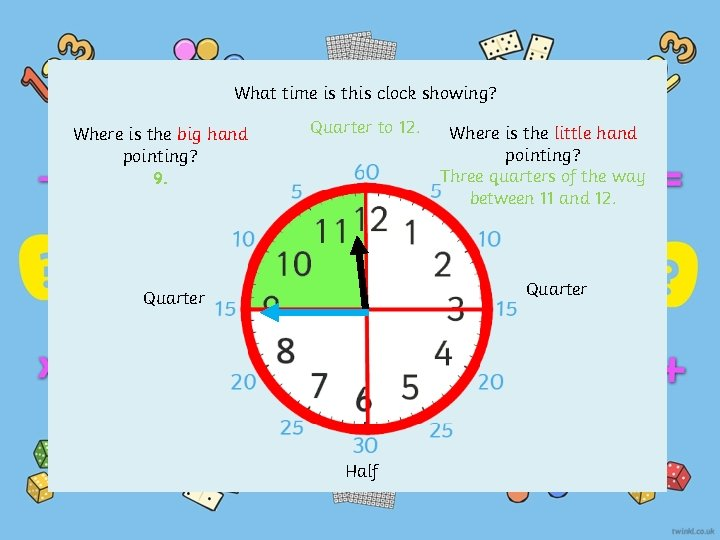What time is this clock showing? Where is the big hand pointing? 9. Quarter to 12. Where is the little hand pointing? Three quarters of the way between 11 and 12. Quarter Half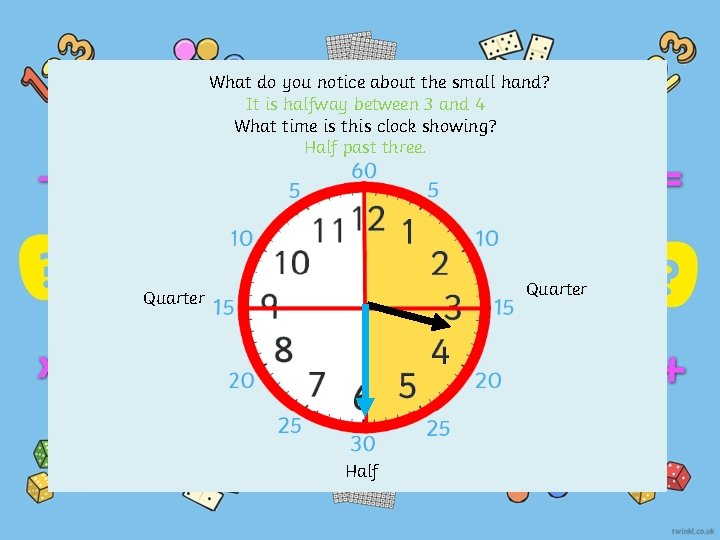What do you notice about the small hand? It is halfway between 3 and 4 What time is this clock showing? Half past three. Quarter HalfPlenary Which clock is showing quarter past? Which clock is showing half past? Which clock is showing quarter to?